# The Ampadu-G Family of Distributions with Application to the T − X (W) Class of Distributions

Department of Biostatistics, USA

Received Date: February 14, 2019;  Published Date: February 22, 2019

#### Abstract

The T − X (W)family of distributions appeared in . In this paper, inspired by the structure of the CDF in the Zubair-G class of distributions , we introduce a new family of distributions called the Ampadu-G class of distributions, and use it to obtain a new class of distributions which we will call the AT − X (W) class of distributions, as a further application of the T − X (W)framework. Sub-models of the Ampadu-G class of distributions and the AT − X (W) class of distributions are shown to be practically significant in modeling real-life data. The Ampadu-G class of distributions is seen to be strikingly similar in structure to the Exponentiated EP (EEP) model contained in , and the Zubair-G class of distributions is seen to be strikingly similar in structure to the Complementary exponentiated Weibull-Poisson (CEWP) model contained in .

Keywords:Zubair-G; T − X (W)family of distributions; Ampadu-G

#### Introduction

##### Background on the T − X (W)family of distributions

Definition 3.1:  Let r (t) be the PDF of a continuous random variable T ∈[a, b] for −∞ ≤ a ≤ b ≤ ∞ and let R(t ) be its CDF. Also let the random variable X have CDF F (x) and PDF f (x) , respectively. A random variable B is said to be T − X (W)distributed if the CDF can be written as the following integral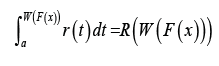whereW (F (x)) satisfies the following conditions

a) W (F (x))∈[a, b]

b) W (F (x))is differentiable and monotonically nondecreasing

c) limx→−∞ W(F(x))= a and limx→−∞ W(F(x))= b

Remark 3.2: By differentiating the RHS of the above equation with respect to x, the PDF

of the T − X (W)family of distributions can be obtained.

Remark 3.3: If the continuous random variable T has support [0, 1], we can take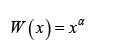where α > 0 . In particular, we will say a random variable B is T − X (W)distributed of type I, if the CDF can be written as the following integral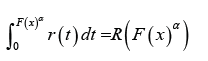Remark 3.4: If the continuous random variable T has support [a,∞)with a ≥ 0we can takeW (x) = −log(1− xα ) orwhere α > 0 . In particular, we will say arandom variable B T − X (W) distributed of type II, if the CDF can be written as either one of the following integrals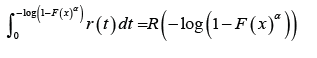OrRemark 3.5: If the continuous random variable T has support (−∞,∞) we can take W ( x) = log(−log(1− xα )) or, where α > 0 . In particular, we will say a random variable B is T − X (W) distributed of type III, if the CDF can be written

as either one of the following integrals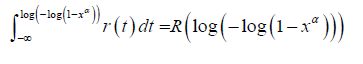or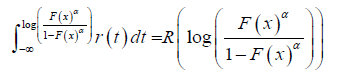Remark 3.6: By differentiating the RHS of the equations in Remark 3.3, Remark 3.4, and Remark 3.5, respectively, we obtain the PDF’s of the class of T − X (W)distributions of type I, II and III, respectively.

##### Background on Zubair-G family of distributions

Definition 3.7:  A random variable B* is said to be Zubair-G distributed if the CDF is given by

where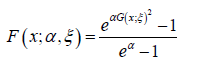Where α ,ξ > 0, x∈R and G is the CDF of the baseline distribution by differentiating the CDF in the above definition we obtain the PDF of the Zubair-G class of distributions as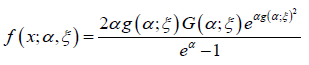Where α ,ξ > 0, x∈R , G is the CDF of the baseline distribution, and g is the PDF of the baseline distribution

#### The New Family of Distributions

##### The Ampadu-G family of distributions

Definition 4.1: Let λ > 0,ξ > 0 be a parameter vector all of whose entries are positive, and x∈R . A random variable X will be said to follow the Ampadu-G family of distributions if the CDF is given by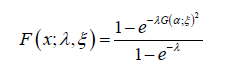and the PDF is given bywhere the baseline distribution has CDF G(x,ξ ) and PDF g(x,ξ )

##### Generalized AT − X (W) Family of Distributions of type I

Definition 4.2: Assume the random variable T with support [0, 1] has CDF G(t;ξ ) and

AT − X (W) distributed of type I if the CDF can be expressed as the following integralWhere λ,ξ ,β > 0, and the random variable X with parameter vector ! has CDF F (x,w) and PDF f (x,w)

Remark 4.3: If β =1 in the above definition we say S is AT − X (W) distributed of type I

##### Generalized AT − X (W) Family of Distributions of type II

Definition 4.4: Assume the random variable T with support [a,∞) has CDF G(t,ξ ) and

PDF g(x,ξ ). We say a random variable S is generalized AT − X (W) distributed of type II if the CDF can be expressed as either one of the following integralsOrwhere λ,ξ ,β > 0 and the random variable X with parameter vector ! has CDF F (x,w) and PDF f (x,w)

Remark 4.5: If β =1 in the above definition we say S is AT − X (W) distributed of type II

##### Generalized AT − X (W) Family of Distributions of type III

Definition 4.6: Assume the random variable T with support (−∞,∞) has CDF G(t;ξ ) and PDF g(t;ξ ) . We say a random variable S is generalized AT − X (W) distributed of type III if the CDF can be expressed as either one of the following integrals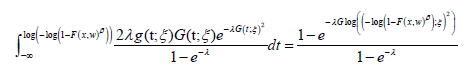orwhere λ,ξ ,β > 0 and the random variable X with parameter vector w has CDF F (x,w) and PDF f (x,w)

Remark 4.7. If β =1 in the above definition, we say S is AT − X (W) distributed of type III

#### Practical Application to Real-life Data

##### Illustration of Ampadu-G family of distributions

We consider the data set  which is on the breaking stress of carbon fibers of 50 mm in length. We assume the baseline distribution is Weibull distributed, so that for x,a,b > 0 , the CDF is given by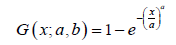and the PDF is given byTheorem 5.1. The CDF of the Ampadu-Weibull distribution is given byWhere x,a,b,λ > 0

Proof. Since the baseline distribution is Weibull distributed, then for x,a,b > 0 , the CDF is given by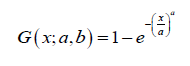So the result follows from Definition 4.1

Remark 5.2: If a random variable R is Ampadu-Weibull distributed, we write R ~ AW (a,b,λ ) (Figure 1).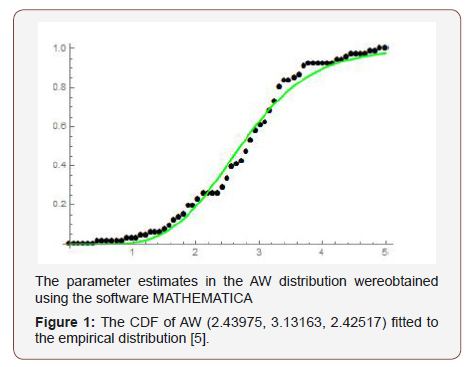##### Illustration of AT − X (W) Family of Distributions of type I

The data set refers to the remission times (in months) of a random sample of 128 bladder cancer patients studied in . We assume the random variable T follows the Burr X (BX) family of distributions so that for t, a, b > 0, the CDF is given by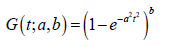and the PDF is given byWe assume the random variable X is Lomax distributed so the for x, c, d > 0, the CDF is given byand the PDF is given byNow we consider Remark 4.3 in Definition 4.2, then we get the following

Theorem 5.3: The CDF of the ABurrX−Lomax family of distributions, for x,a,b,c,d,λ > 0 is given byRemark 5.4: If a random variable W has CDF given by the ABurrX − Lomax family of distributions, we write W  ABXL(a,b,c, d,λ ) (Figure 2).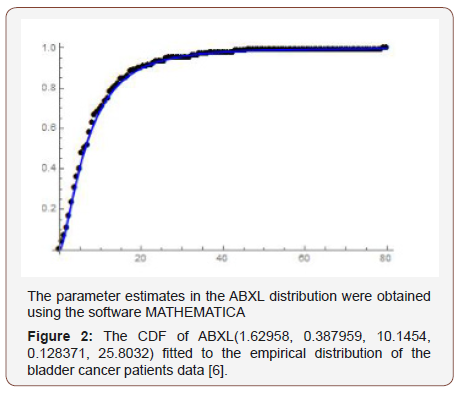##### Illustration of AT − X (W) Family of Distributions of type II

The second data set is on 30 successive March precipitation (in inches) observations obtained from  and recorded in Section 7 of . We assume the random variable T with support [0,∞) follows the Weibull distribution, so that for t > 0, and b, c > 0, the CDF is given by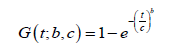and the PDF is given by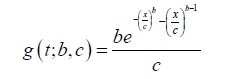We also assume the random variable X follows the Rayleigh distribution, so that for x, a > 0, the PDF is given byand the CDF is given by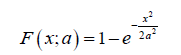Considering Remark 4.5 in the first integral of Definition 4.4, we get the following Theorem 5.5. The CDF of the AWeibull − Rayleigh family of distributions of type II is given by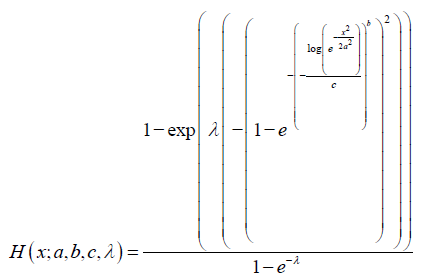Remark 5.6: When a random variable J* has CDF given by Theorem 3.5, we write J* ~ AWR(a,b,c,λ) (Figure 3).##### Illustration of AT − X (W) Family of Distributions of type III

In this application we consider the data set in  from , on the breaking stress of carbon fibers of 50 mm in length. We assume the random variable T with support (−∞,∞) follows the Cauchy distribution, so that for t, a 2 R, b > 0, the CDF is given by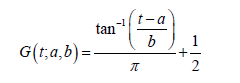and the PDF is given byWe also assume the random variable X follows the Weibull distribution, so that for x > 0, and c, d > 0, the CDF is given byand the PDF is given by now considering Remark 4.7 in the second integral of Definition 4.6, we get the following

Theorem 5.7: The CDF of the ACauchy − Weibull family of distributions of type III is given by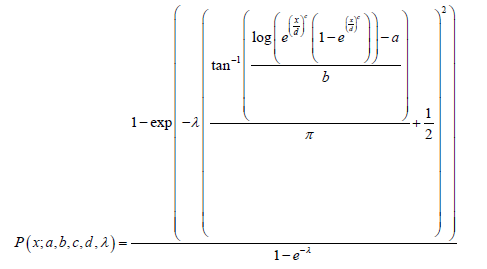Where x;a,b,c,d,λ > 0

Remark 5.8: By differentiating the CDF of the ACauchy−Weibull family of distributions of type III, the PDF can be obtained

Remark 5.9: When a random variable N* has CDF given by Theorem 5.7, we write (Figure 4) N* ~ ACW (a,b,c,d,λ)None.

#### Conflict of Interest

No conflict of interest.

Article Details
Citation
Keywords
Scroll to
Scroll to Top